# The reaction of Cr203 with silicon metal at high temperatures will make chromium metal. 2Cr,03 (s)...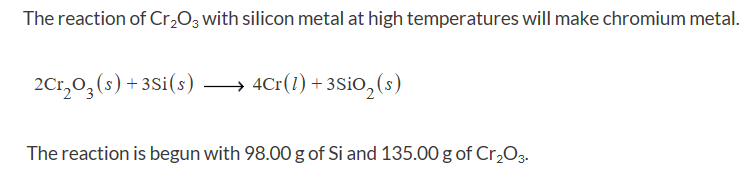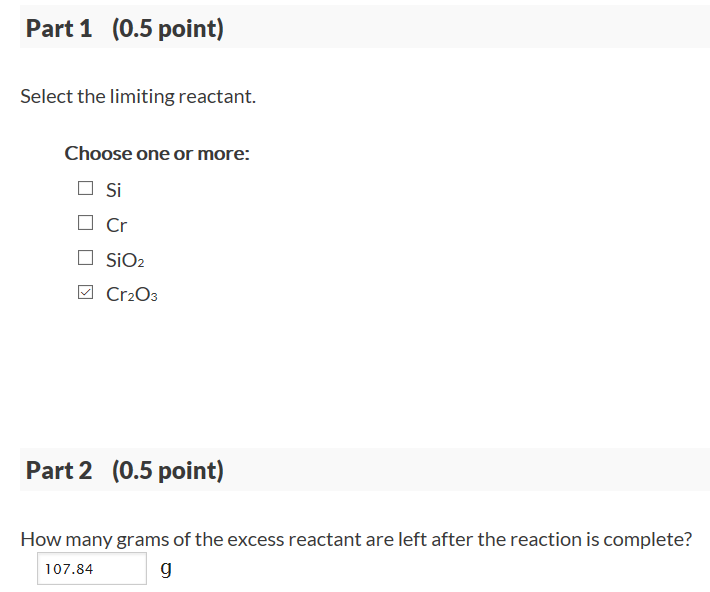The reaction of Cr203 with silicon metal at high temperatures will make chromium metal. 2Cr,03 (s) +3Si(s) → 4Cr(1) +3SiO2 (s) The reaction is begun with 98.00 g of Si and 135.00 g of Cr203

1)

Molar mass of Cr2O3 = 2*MM(Cr) + 3*MM(O)

= 2*52.0 + 3*16.0

= 152 g/mol

mass of Cr2O3 = 135.0 g

we have below equation to be used:

number of mol of Cr2O3,

n = mass of Cr2O3/molar mass of Cr2O3

=(135.0 g)/(152 g/mol)

= 0.8882 mol

Molar mass of Si = 28.09 g/mol

mass of Si = 98.0 g

we have below equation to be used:

number of mol of Si,

n = mass of Si/molar mass of Si

=(98.0 g)/(28.09 g/mol)

= 3.489 mol

we have the Balanced chemical equation as:

2 Cr2O3 + 3 Si ---> 4 Cr + 3 SiO2

2 mol of Cr2O3 reacts with 3 mol of Si

for 0.8882 mol of Cr2O3, 1.332 mol of Si is required

But we have 3.489 mol of Si

so, Cr2O3 is limiting reagent

2)

we will use Cr2O3 in further calculation

From balanced chemical reaction, we see that

when 2 mol of Cr2O3 reacts, 3 mol of Si reacts

mol of Si reacted = (3/2)* moles of Cr2O3

= (3/2)*0.8882

= 1.332 mol

mol of Si remaining = mol initially present - mol reacted

mol of Si remaining = 3.489 - 1.332

mol of Si remaining = 2.157 mol

Molar mass of Si = 28.09 g/mol

we have below equation to be used:

mass of Si,

m = number of mol * molar mass

= 2.157 mol * 28.09 g/mol

= 60.6 g

Feel free to comment below if you have any doubts or if this answer do not work. I will edit it if you let me know

##### Add Answer to: The reaction of Cr203 with silicon metal at high temperatures will make chromium metal. 2Cr,03 (s)...
Similar Homework Help Questions
• ### The reaction of Cr203 with silicon metal at high temperatures will make chromium metal. 2Cr,0(s) ...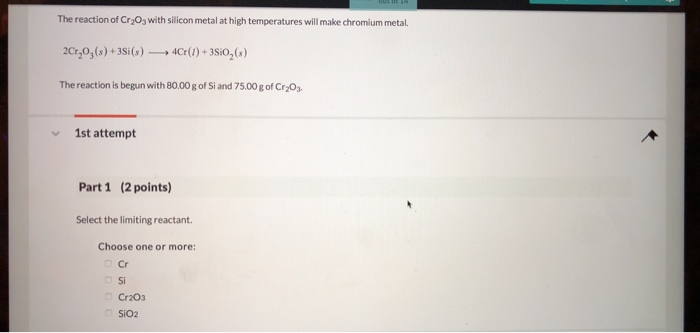The reaction of Cr203 with silicon metal at high temperatures will make chromium metal. 2Cr,0(s) +3Si(s)4r()+3Sio2(s) The reaction is begun with 80.00g of Si and 75.00 g of Cr203 1st attempt Part 1 (2 points) Select the limiting reactant. Choose one or more: Cr Si Cr203 Si02 Cr203 SiO2 Part 2 (2 points) How many grams of the excess reactant are left after the reaction is complete? K02/04> 0 OF 4 QUESTIONS COMPLETED MacBook 딤0 2 3 4 6 The...

• ### The reaction of Cr2O3 with silicon metal at high temperatures will make chromium metal. 2Cr2O3​(s) +...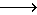The reaction of Cr2O3 with silicon metal at high temperatures will make chromium metal. 2Cr2O3​(s) + 3Si(s) 4Cr(l) + 3SiO2(s) The reaction is begun with 188.00 g of Si and 110.00 g of Cr2O3. A) What is the limiting reactant? B) How many grams of the excess reactant are left after the reaction is complete?

• ### The reaction of Cr2O3 with silicon metal at high temperatures will make chromium metal. 2Cr,0,(s) +...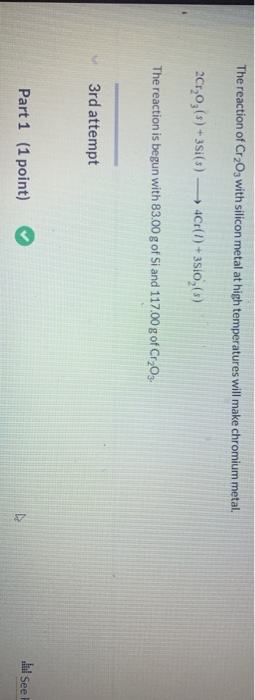The reaction of Cr2O3 with silicon metal at high temperatures will make chromium metal. 2Cr,0,(s) + 3Si(s) — 4Cr(I) +3510,(s) The reaction is begun with 83.00 g of Si and 117.00 g of Cr2O3. 3rd attempt Part 1 (1 point) l See Part 2 (1 point) * Feedback How many grams of the excess reactant are left after the reaction is complete? 100.8 2nd attempt 1st attempt

• ### The reaction of Cr2O3 with silicon metal at high temperatures will make chromium metal. 2CrO3(s)+3Si(s)---> 4Cr(l)+3SiO2(s)...

The reaction of Cr2O3 with silicon metal at high temperatures will make chromium metal. 2CrO3(s)+3Si(s)---> 4Cr(l)+3SiO2(s) The reaction is begun with 107.00g of Si and 115.00g of Cr2O3. How many grams of the excess reactant are left after the reaction is complete?

• ### The reaction of Cr_2O_3 with silicon metal at high temperatures will make chromium metal. 2Cr_2O_3 (s)...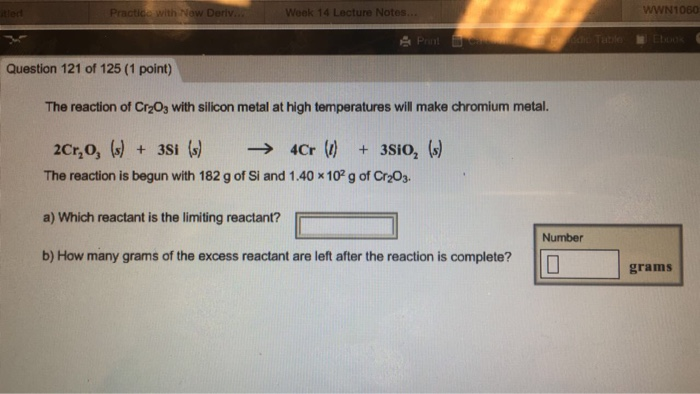The reaction of Cr_2O_3 with silicon metal at high temperatures will make chromium metal. 2Cr_2O_3 (s) + 3Si (s) rightarrow 4Cr (l) + 3SiO_2 (s) The reaction is begun with 182 g of Si and 1.40 times 10^2 g of Cr_2O_3. Which reactant is the limiting reactant? How many grams of the excess reactant are left after the reaction is complete?

• ### 16 Question (1 point) The reaction of Cr2O3 with silicon metal at high temperatures will make...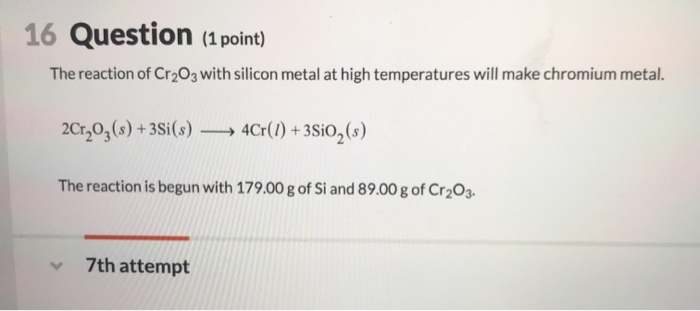16 Question (1 point) The reaction of Cr2O3 with silicon metal at high temperatures will make chromium metal. 2Cr,0g(s)+3Si(s) 4Cr()+3Sio, (s) The reaction is begun with 179.00 g of Si and 89.00 g of Cr2O3 7th attempt (0.5 point) Part 2 How many grams of the excess reactant are left after the reaction is complete?

• ### 20 Question (2 points) Chromium metal can be produced from the high temperature reaction of Cr2O3 [chromium(lII) oxide]...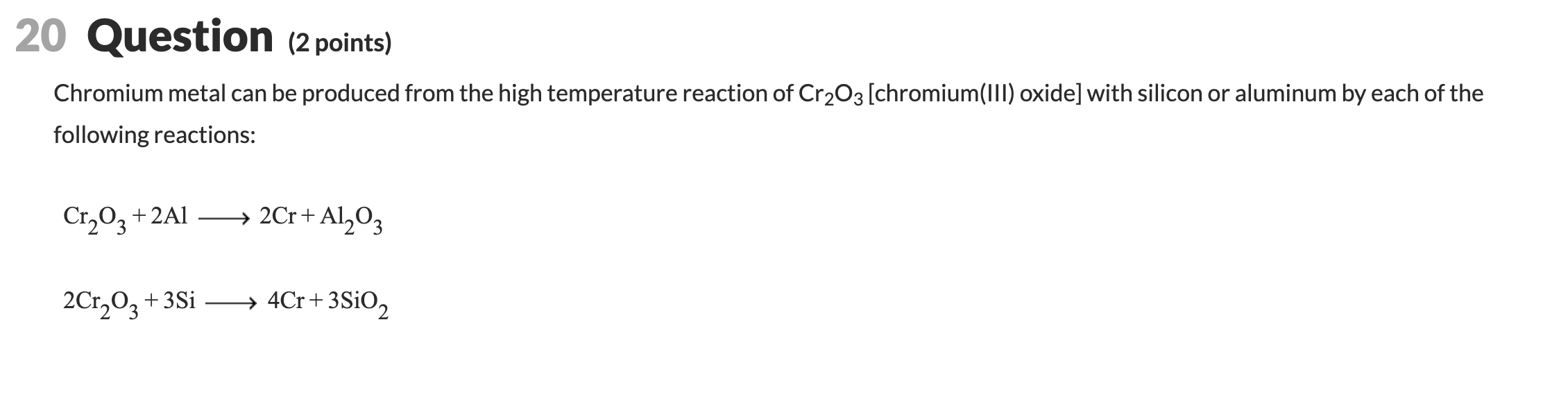20 Question (2 points) Chromium metal can be produced from the high temperature reaction of Cr2O3 [chromium(lII) oxide] with silicon or aluminum by each of the following reactions: 2Cr+ Al203 Cr203+2A 4Cr 3SIO2 2Cr203+3Si See Periodic Table (1 point) xFeedback Part 1 Calculate the number of grams of aluminum required to prepare 195.0 g of chromium metal by the first reaction. 169.7 See Hint (1 point) Part 2 X Calculate the number of grams of silicon required to prepare 195.0...

• ### 16 Question (1 point) The reaction of Cr2O3 with silicon metal at high temperatures will make...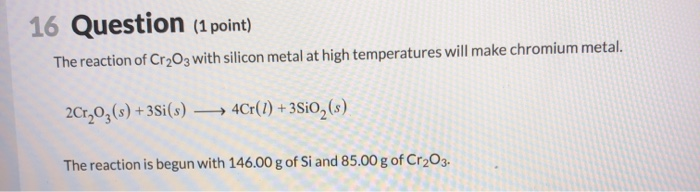16 Question (1 point) The reaction of Cr2O3 with silicon metal at high temperatures will make chromium metal. 2Cr2O3(s) +35i(s) —— 4Cr(1) + 3Si0, (s) The reaction is begun with 146.00 g of Si and 85.00 g of Cr2O3. Part 2 (0.5 point) How many grams of the excess reactant are left after the reaction is complete?

• ### 21 Question (1 point) Chromium(III) oxide reacts with silicon as indicated by the balanced equation below. 2Cr,0, (s) +...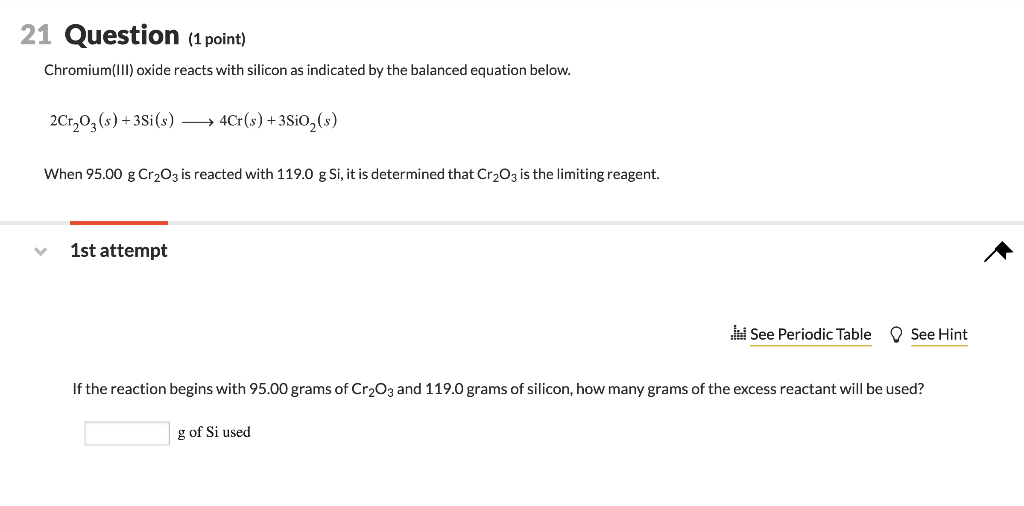21 Question (1 point) Chromium(III) oxide reacts with silicon as indicated by the balanced equation below. 2Cr,0, (s) +35i(s) — 4Cr(s) +3Si0,(s) When 95.00 g Cr203 is reacted with 119.0 g Si, it is determined that Cr2O3 is the limiting reagent. 1st attempt . See Periodic Table See Hint If the reaction begins with 95.00 grams of Cr2O3 and 119.0 grams of silicon, how many grams of the excess reactant will be used? g of Si used

• ### Calculate the number of moles of aluminum and silicon required to produce 199.0 g of chromium based on the following equ...Calculate the number of moles of aluminum and silicon required to produce 199.0 g of chromium based on the following equations: Cr2O3 + 2Al 2Cr + Al2O3 2Cr2O3 + 3Si 4Cr + 3SiO2 We were unable to transcribe this imageWe were unable to transcribe this image

Free Homework App